Create a new printable
Answer key also includes questions
Answer key only gives the answers
No answer keyMath Worksheets
Multiplication

Sample - Click above to make a new math worksheet (PDF).
 Name _____________________________Date ___________________
Addition and Multiplication
Complete the addition and multiplication fact.

1.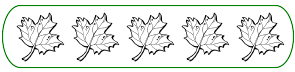___ + ___ = ______ ___ x ___ = ______
2.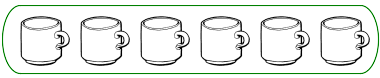___ + ___ + ___ = ______ ___ x ___ = ______
3.___ + ___ = ______ ___ x ___ = ______
4.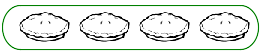___ + ___ + ___ + ___ = ______ ___ x ___ = ______
5.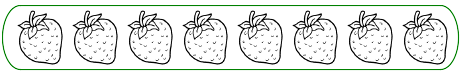___ + ___ = ______ ___ x ___ = ______
6.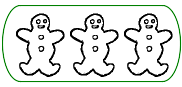___ + ___ + ___ + ___ + ___ + ___ = ______ ___ x ___ = ______
7.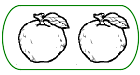___ + ___ + ___ + ___ + ___ + ___ + ___ + ___ = ______ ___ x ___ = ______
8.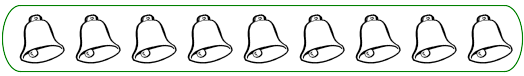___ + ___ = ______ ___ x ___ = ______
 9 * This is a pre-made sheet.Use the link at the top of the page for a printable page.

 Answer Key

Sample
This is only a sample worksheet.

Create a new printable
Answer key also includes questions
Answer key only gives the answers
No answer keyMath Worksheets
Multiplication